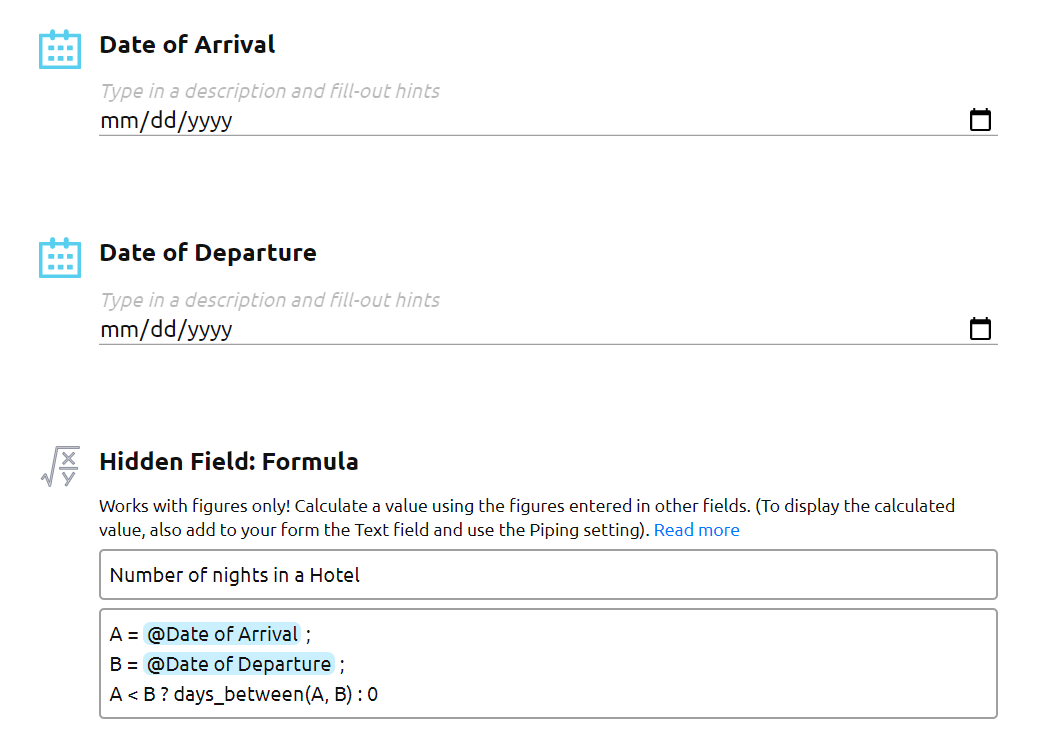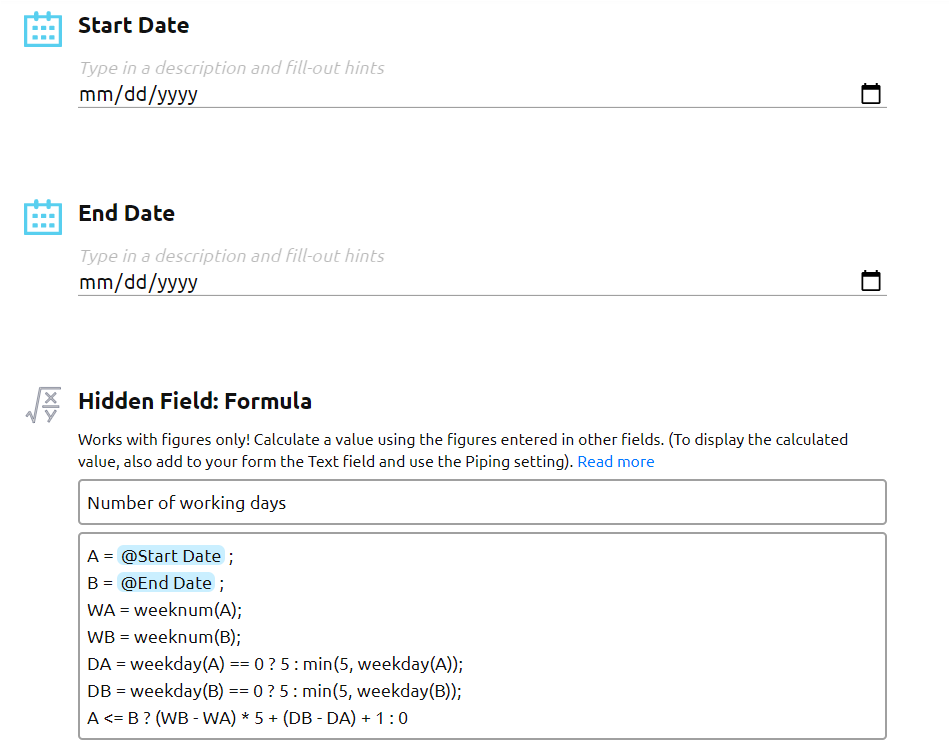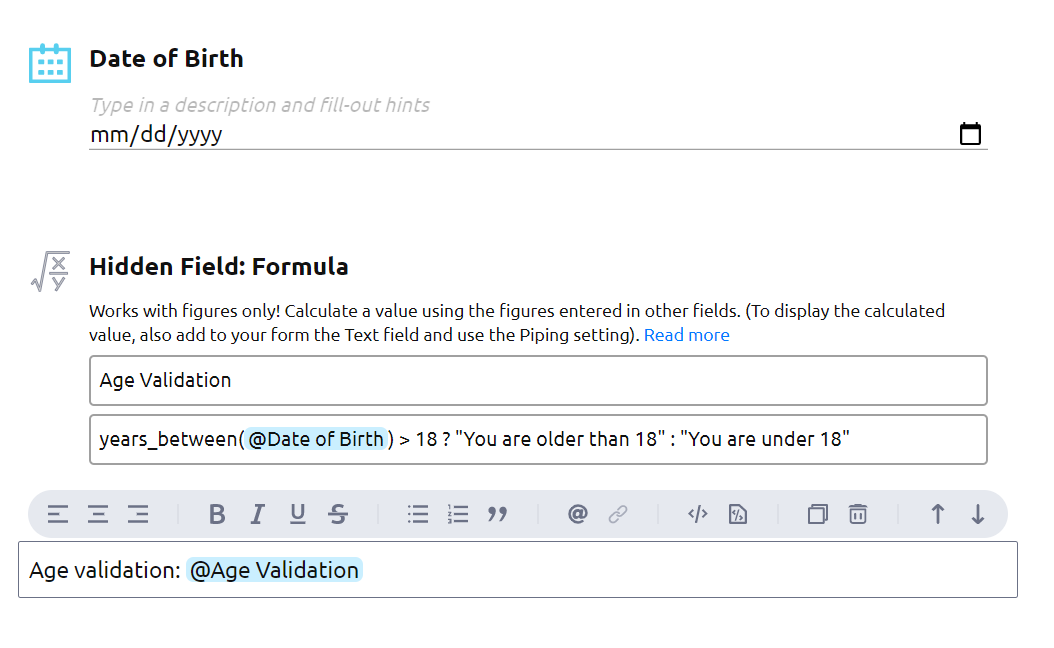# General information About the Date field. How to Use the Date Fields in Formulas

The Date field is located in the Special section of the Form Elements panel. The field allows you to collect data about the date that your respondent enters or chooses using the date picker extension on the form.

The date displayed on the published form is formatted based on the locale of the user’s browser.

The parsed value — the date that you will see on the PDF reports, in CSV/Excel/Google Sheet documents, is always formatted yyyy-mm-dd.

The Date field can be used in the Formula field.

When you add a Date field in a Formula field using mentions (@), its value is converted into the Unix timestamp.

Presently, AidaForm has the following functions for the Date fields that will help you easily make different calculations:

• now() - returns the current date in the Unix timestamp;
• days_between(a, b) - calculates and returns the number of days between the values of Date fields a and b;
• days_between(a) - calculates and returns the number of days between the values of the Date a and the current date;
• years_between(a,b) - calculates and returns the round number of years between the values of Date fields a and b;
• years_between(a) - calculates and returns the round number of years between the values of the Date a and the current date;
• weekday(a) - returns the day of the week for the Date a. 0 - Sunday, 1 - Monday, 6 - Saturday;
• weeknum(a) - returns the week number of a specific date.

## Examples of how to use the Date fields in Formula fields to calculate:

1. The number of days/nights between two dates
2. The number of working days between two dates
3. The age of your respondent (in years)
4. If your respondent has reached the specified age

Read how the Formula field works here

## Example 1:

Your form has two Date fields: Date of Arrival and Date of Departure. You would like to calculate the number of days/nights between these dates.

The formula may look like this:

A = @Date of Arrival ;
B = @Date of Departure ;
A < B ? days_between(A, B) : 0

If the Date of Arrival is before the Date of Departure, then the formula counts the number of days between the dates, if not, the number is equal to 0. You may use the result in other Formula fields on your form, for example, to calculate the total cost of a stay in a hotel.## Example 2:

Your form has two Date fields: Start Date and End Date. You would like to calculate the number of working days (Monday-Friday) between these dates.

The formula may look like this:

A = @Start Date ;
B = @End Date ;
WA = weeknum(A);
WB = weeknum(B);
DA = weekday(A) == 0 ? 5 : min(5, weekday(A));
DB = weekday(B) == 0 ? 5 : min(5, weekday(B));
A <= B ? (WB - WA) * 5 + (DB - DA) + 1 : 0## Example 3:

Your form has the field Date of Birth and you would like to calculate the age of your respondent.

The formula may look like this:

years_between(@Date of Birth)

## Example 4:

Your form has the field Date of Birth and you would like to calculate whether your respondent is older or younger than 18.

The formula may look like this:

years_between(@Date of Birth) > 18 ? "You are older than 18 " : "You are under 18"

The formula calculates the difference in years between the current moment and the date of birth, and if the respondent is older than 18 years old, it returns the text ‘You are older than 18’, otherwise it returns the text ‘You are under 18’.Does your use case differ from those in the examples? Are you not sure how to make calculations in your case? Use this form to describe your case and we will get back to you with a possible solution!